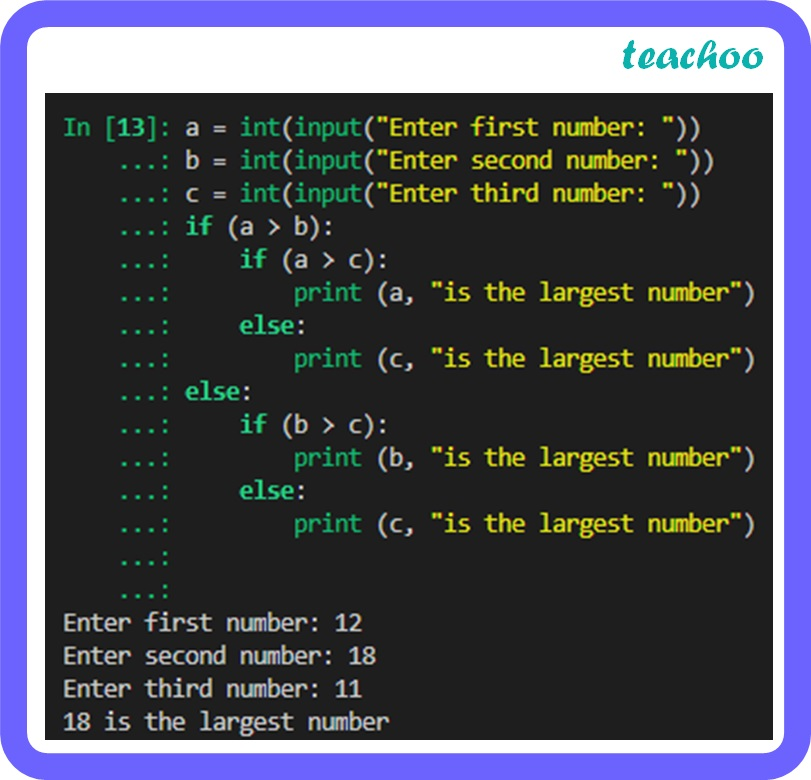Long Answer Type Questions (5 Marks each)

Computer Science - Class 11
Chapter 6 Class 11 - Flow of Control

## Write a program to find the largest number among the three given numbers.

Code:

``` a = int(input( "Enter first number: " )) ```

``` b = int(input( "Enter second number: " )) ```

``` c = int(input( "Enter third number: " )) ```

``` if (a > b):  ```

```     if (a > c):  ```

```         print (a, "is the largest number" )  ```

```     else:  ```

```         print (c, "is the largest number" )  ```

``` else:  ```

```     if (b > c):  ```

```         print (b, "is the largest number" )  ```

```     else:  ```

```         print (c, "is the largest number") ```Learn in your speed, with individual attention - Teachoo Maths 1-on-1 Class